### Home > CALC > Chapter 5 > Lesson 5.1.1 > Problem5-7

5-7.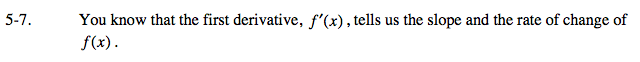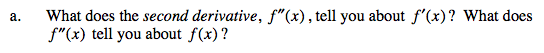f ''(x) tells us the same thing about f '(x) that f '(x) tells us about f(x).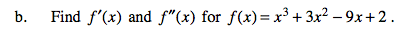f '(x): 3x² + 6x −9
f ''(x): 6x + 6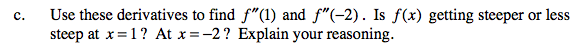Consider this: Even though a slope of −2 is steeper than a slope of −1, if the slope changes from −1 to −2, then the slope is decreasing.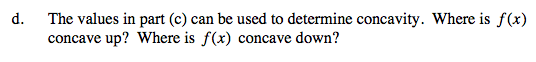Positive values of the 2nd-derivative indicate that the function is concave up while negative values indicate that the function is concave down.